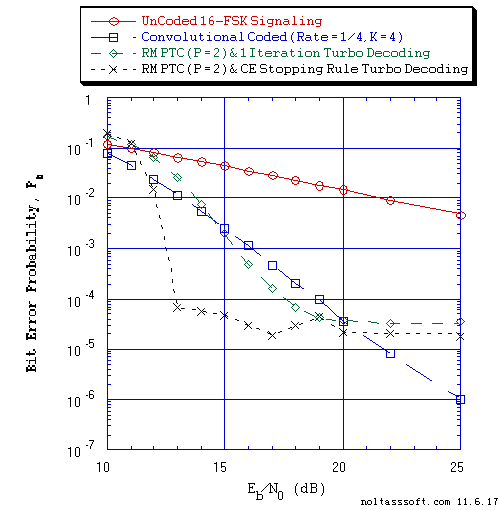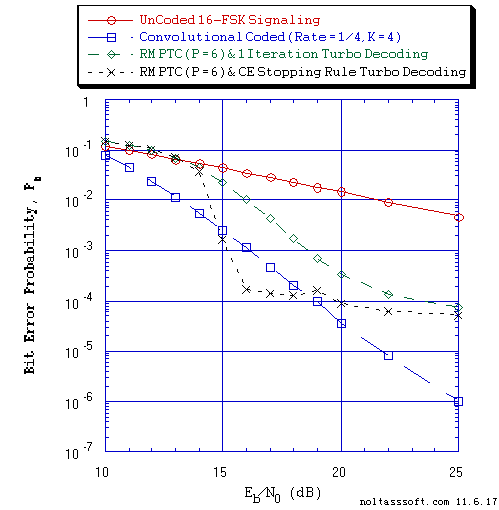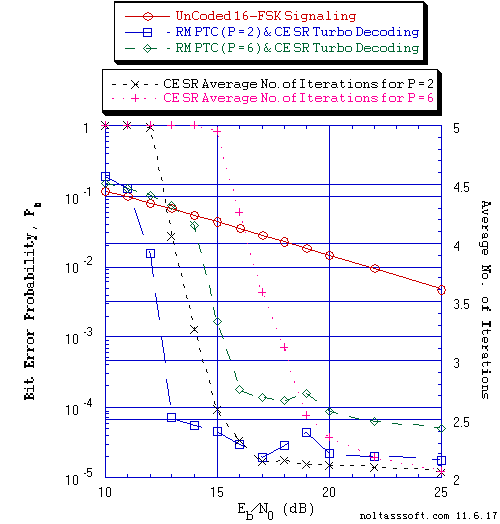# RATE MATCHING PUNCTURED TURBO CODED SIGNALING over a MEMORY CHANNEL

NOTE: This section is Under-Edit if necessary: Construction began on November 14, 2017 and was finished on November 14, 2017.

Turbo Coded Signaling over a Memory Channel: Rate Matching, Rayleigh Fading, and the Iterative Turbo Decoder

```by Darrell A. Nolta
November 8, 2017
```

The AdvDCSMT1DCSS (T1) Professional (T1 Version 2) system tool now offers the capability to model and simulate Rate Matching (RM) Punctured Turbo Coded Signaling over Memory Channel (MemC) with Additive White Gaussian Noise (AWGN). This feature is in addition to T1 V2's capability to model and simulate Turbo Coded (TC) and Punctured Turbo Coded (PTC) Signaling over MemC.

T1 V2 provides the option with this RM Signaling feature to use Multiple Iteration Soft Input/Soft Decision Output (SISO) TC Channel Decoding using a Punctured Turbo Decoding Algorithm where two SISO 'symbol-by-symbol' Maximum a Posteriori Probability (MAP) Algorithm component decoders (DEC1 & DEC2) are serial concatenated with a feedback loop.

In addition, T1 Professional provides the option with this RM Signaling feature to model and simulate a Non-Iterative (Single Iteration) SISO PTC Channel Decoding where DEC1 and DEC2 are serial concatenated without the feedback loop.

This capability of T1 Professional supports decoding of Rate Matching Punctured Turbo Coded M-ary Signaling over a Gilbert-Elliot (GE) Burst Channel, Fading Channel (Rayleigh, Rician, and Frequency-Selective), or Intersymbol Interference (ISI) Channel.

T1 V2 provides the User the ability to add a Symbol Interleaver-DeInterleaver for the GE Burst Channel or Fading Channel Signaling simulations. In a Fading Channel simulation, a Diversifier-Combiner can be used instead of the Symbol Intlerleaver-DeInterleaver. In an ISI Channel simulation, a Linear Equalizer can be added.

The Rate Matching Signaling requires that a parent Turbo Code is punctured so that the Punctured Turbo Code Rate is increased. One can achieve a Punctured Rate Rp of 1/2, 2/3, 3/4, 2/5, or 3/5 for a parent TC Rate of 1/3; Rp of 1/2, 2/3, 3/4, 2/5 or 3/5 for a parent TC Rate of 1/5; and Rp of 1/2, 2/3, 3/4, 2/5, or 3/5 for a parent TC Rate of 1/7.

Also, this capability of T1 Professional supports decoding of RM PTC Signaling over a Classic Bursty Channel (CBC) with background error. In a CBC simulation, a Block Interleaver-DeInterleaver can be used.

This paper will focus on the application of T1 V2 in the study of the use of Turbo Coding and Decoding to reduce the Information Bit Errors caused by Rayleigh Fading. Rayleigh Fading is a very important channel impairment that is very destructive in a wireless/mobile communication system that attempts to signal over a dispersive (multipath) environment.

Rayleigh fading channel is a flat (non-selective) fading channel whose parameters vary in time that applies a multiplicative complex Gaussian noise on the transmitted signal. The Rayleigh Fading Channel is a Memory Channel where the physical state of this channel can be characterized by the fading level of a slowly fading transmission path (Robert G. Gallager , page 97). It is important to realize that this channel does not act on each of its inputs in a statistically independent manner. This channel is destructive of the phase information contained within the symbol-to-symbol detection of the channel output signal (i.e., phase variation of the transmitted/received signal). Coherent detection/demodulation cannot be use as long as this phase information cannot be recovered from the received signal.

Given the nature of the Rayleigh Fading Channel, the Frequency Shift Keying (FSK), an orthogonal modulation, is the only modulation to be use for this channel as long as noncoherent demodulation is the only possibility and if the required signal bandwidth is not a limiting factor.

Thus, T1 V2 will offer only FSK modulation when the User selects the Fading Channel for a simulation.

A Soft Bit Metric is used by the Rate Matching Punctured Turbo Decoder when an M > 2 Modulation Scheme is used for signaling over an AWGN Memory Channel. A Demodulation Constellation DeMapper will produce a Max-Log or Log-Sum Soft Bit Metric (User Specified).

And, for the Fading Channel selection, the Turbo Decoder uses Energy Detector Demodulation with Fading Statistics.

Consider the Rate Matching (RM) Punctured Turbo Decoding Algorithm Bit Error Rate (BER) or Bit Error Probability Pb performance simulation results that were produced by T1 V2 that are displayed below in Figure 1, 2, and 3 plots. Also, in addition to the Pb results, Figure 3 displays the corresponding Average Number of Iterations per Block (Avg I).

Note that the BER results are based on the use of an Equal Probable Independent and Identically Distributed (i.i.d.) Source.

For comparison purposes, simulated BER results for UnCoded and Convolutional Coded (CC) (Rate = 1/4, 16-State) 16-FSK Signaling are included appropriately in these figures.

The Code Rate = 1/4, Constraint Length K = 4 convolutional code is a maximal minimum free distance code (a S. Lin and D.J. Costello, Jr. code) as described by Stephen B Wicker , page 284.

Maximum Likelihood (ML) Energy Detector Demodulation without Fading Statistics is used for UnCoded 16-FSK Signaling. And ML Energy Detector Demodulation without Fading Statistics and Viterbi Algorithm Decoding is used for CC 16-FSK Signaling.

For Rate Matching Punctured Turbo Coded 16-FSK Signaling, a Turbo Code Rate (r) = 1/3, K = 4 using a r = 1/2, K = 4 Optimum-weight Spectrum Recursive Systematic Convolutional (RSC) code is used along with a 6144-Bit QPP Interleaver.

Each figure's BER plot displays three or more curves where a curve is constructed from the set of simulated Pb values that correspond to a set of Eb/N0 [Signal-to-Noise Ratio (SNR)] values in dB. Each Rate Matching Punctured Turbo Coding (RM PTC) curve displays the performance behavior of a RM PTC and Iterative Decoding system example that was used for a T1 V2 simulation.

Figure 1 displays the BER versus Eb/N0 for One Iteration and Cross-Entropy (CE) Stopping Rule (SR) Iterative Turbo Decoding of Punctured Turbo Coded Rate = 1/2 Rate Matching Punctured Turbo Coded 16-FSK Signaling for 1,001,472 Equal probable i.i.d. Information (Info) Bits and Puncturing Period (P) of 2.

Figure 2 displays the BER versus Eb/N0 for One Iteration and CE Stopping Rule Iterative Turbo Decoding of Punctured Turbo Coded Rate = 3/4 Rate Matching Punctured Turbo Coded 16FSK Signaling for 1,001,472 Equal probable i.i.d. Info Bits and Puncturing Period (P) of 6.

Figure 3 displays BER and Avg I versus Eb/N0 for CE Stopping Rule Iterative Turbo Decoding of Rate = 1/2 and Rate = 3/4 Rate Matching Punctured Turbo Coded 16-FSK Signaling for 1,001,472 Equal probable i.i.d. Info Bits.

There are a number of important observations that can be drawn from the below displayed simulated Iterative Rate Matching Punctured Turbo Code (IPTC) Decoding BER results. It appears that T1 is correctly modeling and simulating Rate Matching Punctured Turbo Coded Signaling over a Rayleigh Fading Channel (Memory Channel) with AWGN with Iterative Decoding for the selected Punctured Turbo Code.

In Figure 1 or 2, we contrast the BER performance of Iterative Turbo Decoding for RM Punctured Turbo Coded 16-FSK Signaling using a Puncturing Period (P) of two or six, respectively, against the BER performance of UnCoded and CC 16-FSK Signaling. In Figure 3, we contrast the BER performance and Avg I for P of two and six cases.

We can clearly observe in Figure 1 the dramatic reduction in BER (~1.3x10-1 to ~7.1x10-5) in the low SNR region (known as the 'waterfall') for the RM PTC 16-FSK Signaling with IPTC Decoding [CE Stopping Rule (SR)] as compared to the UnCoded 16-FSK or CC 16-FSK Signaling with Viterbi Algorithm (VA) Decoding or RM PTC One Iteration IPTC Decoding. After reaching the waterfall BER minimum, the BER curve begins its oscillation as SNR increases (13 dB to 25 dB). Eventually, the BER reaches ~1.8x10-5 at SNR equal to 25 dB. In regards to the One Iteration IPTC Decoding BER curve, the BER decreases gracefully with increasing SNR and then levels off. In the 'high SNR' region, the BER values corresponding to the CE SR IPTC Decoding are less than the BER values corresponding to the One Iteration ITC Decoding except for the one at SNR value of 19 dB. And at 'high SNR', CC 16-FSK Signaling and VA Decoding BER values are less than the IPTC Decoding of RM PTC 16-FSK Signaling for SNR values greater than 21 dB.

In Figure 2 [RM PTC (P = 6) 16-FSK Signaling with IPTC Decoding], we can see the same BER behavior as seen in Figure 1 except the CE SR IPTC Decoding curve 's 'waterfall' BER minimum is larger (~1.8x10-4 at 16 dB vs. ~7.1x10-5 at 13 dB). And the 'waterfall' region shifts to larger SNR values (14 - 16 dB as compared to 11 - 13 dB).

In Figure 3 [CE SR IPTC Decoding for Puncturing Period (P) of 2 and 6 and RM PTC 16-FSK Signaling], we can view that the 'waterfall' BER segment occurs when the Average Number of Iterations (Avg I) drops from 5 to ~4.1 ('waterfall' BER minimum occurs at SNR equal to 13 dB) for P of 2. The Avg I plummets from ~4.1 to ~2.3 at SNR equal to 16 dB. For P = 6, we can view that the 'waterfall' BER segment occurs when the Average Number of Iterations (Avg I) drops from 5 to ~4.3 ('waterfall' BER minimum occurs at SNR equal to 16 dB). The Avg I plummets from ~4.3 to ~2.5 at SNR equal to 19 dB.

Based on these BER results displayed in Figures 1, 2, and 3, the BER behavior of Turbo Decoding of Rate Matching Punctured Turbo Coded Signaling over a Rayleigh Fading Channel (a Memory Channel) with AWGN appears to exhibit the known BER behavior of Turbo Decoding of Turbo Coded/Punctured Turbo Coded Signaling over a Memory Channel (MemC) with AWGN [i.e., as the number of iterations is increased (from one iteration to multiple iterations), the SNR corresponding to the 'waterfall' BER minimum is reduced (i.e., moving toward the Shannon Limit)].

But how is this possible?

As a reminder, let us review the basic underlying algorithm applied by each component decoder (DEC1 & DEC2) of a Turbo Decoder. This algorithm is based on the BCJR algorithm that is described by L.R. Bahl, J. Cocke, F. Jelinek, & J. Raviv. in their 1974 paper . Each component decoder implements a SISO 'symbol-by-symbol' Maximum a Posteriori Probability (MAP) Algorithm. Each component decoder's MAP Algorithm calculates the a posteriori probability L-value (natural log likelihood ratio) for each Information Bit. The Turbo Decoder's component MAP decoder is using a metric that is based on sums and not on products of likelihood ratios of a memoryless channel (MLC) representation,

The purpose of the BCJR algorithm is to estimate optimally the a posteriori probability (APP) of each Information Bit that is transmitted. It is important to realize that the BCJR algorithm is based on the definition that the probability of a particular channel output sequence is a product of individual transition probabilities, i.e., these individual transition probabilities are statistically independent of each other. A MLC satisfies this definition. An AWGN channel is a MLC. The memory channel does not satisfy this definition: the transition probabilities are not statistically independent and, thus, the product of these individual probabilities does not represent the channel output sequence probability.

The Rayleigh Fading Channel without AWGN is a Memory Channel. When AWGN is added to the Rayleigh Fading Channel, the channel output would appear to become statistically independent. This fact may explain why Turbo Decoding achieves such good BER results for the Rayleigh Fading Channel with AWGN. We must observe the fact that it is impossible practically to transmit information over a Rayleigh Fading Channel without AWGN. But these BER results are not optimal because the Turbo Decoder (serial concatenated component decoders with a feedback loop) is not an optimal decoder and the Non-Coherent Demodulator does not take into consideration the Markov State structure of the Rayleigh Fading Channel.

T1 Professional (T1 V2) now offers the Turbo Channel Coding and Turbo Channel Decoding (Iterative & Non-Iterative; based on the 'Symbol-by-Symbol' MAP algorithm) for Memory Channel features to the User. One can choose to puncture the Turbo Code. The User can choose the simulated Rate Matching (RM) Signaling Channel as the Classic Bursty Channel (CBC) with background error or Gilbert-Elliot Burst Channel (GEBC); Rayleigh, Rician, or Frequency-Selective Fading Channel; or PAM or QAM InterSymbol Interference Channel with Linear Equalizer. A Block Interleaver-DeInterleaver (Intl-DeIntl) can be used with CBC or a Symbol Intl-DeIntl can be used with GEBC or Fading Channel. A Diversifier -Combiner can be used for a simulation of RM Signaling over a Fading Channel.

In conclusion, the User via T1 V2 can get experience with these algorithms as applied to Iterative Decoding in simulated digital communication systems for Spacecraft and Mobile Communications Turbo Coding applications.

References:

 Robert G. Gallager, Information Theory and Reliable Communication, John Wiley & Sons, New York, 1968.

 Stephen B. Wicker, Error Control Systems for Digital Communication and Storage, Prentice Hall, Englewood Cliffs, NJ, 1995.

 L.R. Bahl, J Cocke, F. Jelinek, and J. Raviv, "Optimal Decoding of Linear Codes for Minimizing Symbol Error Rate," IEEE Transactions on Information Theory, Vol. 20, pp. 284-287, March 1974.```Figure 1. Bit Error Probability for UnCoded, Convolutional, and Rate Matching
Punctured Turbo Coded (RM PTC) 16-FSK Signaling over a Rayleigh Fading
Memory Channel with Additive White Gaussian Noise (AWGN):
```
```          Equal probable Independent and Identical Distributed  (IID) Source for
10 Million, 1 Million, and 1,001,472 Information Bits for UnCoded,
Convolutional Coded, and RM PTC 16-FSK Signaling respectively over a
Vector Channel;
```
```          Rate = 1/4, Constraint Length K = 4, (15,9,7,15), a Maximal Minimum Free
Distance Non-Recursive Convolutional Code (S. Lin & D.J. Costello, Jr.)
and Viterbi Algorithm using a Path Memory Length of 20 bits and an
Unquantized Branch Metric;
```
```          Rate (r) = 1/3, K = 4 Turbo Code based on a r = 1/2, K = 3 (G0 = 13 octal,
G1 = 17 octal) Recursive Systematic Convolutional component code
(Optimum-Weight Spectrum) and a 6144-Bit QPP Turbo Encoder Interleaver;
```
```          Punctured Turbo Code Rate = 1/2 for Puncturing Period of 2 and Puncturing
Matrix P = [[1,1],[1,0],[0,1]];
```
```          Rayleigh Fading Channel: Received Information Baseband SNR Loss due to
```
```          16-FSK Demodulation Constellation DeMapper Algorithm: Max-Log Bit Metrics
& ML Energy Detector; and
```
```          Max-Log-MAP Iterative Turbo Decoder using Systematic Channel Output as
input to both component decoders and Decode Information Bit Decision is
based on the Sum of both decoders' log-likelihood ratios (L-values) and
for One Iteration and Cross-Entropy (CE) Stopping Rule (Five Iterations
Maximum).
``````Figure 2. Bit Error Probability for UnCoded, Convolutional, and Rate Matching
Punctured Turbo Coded (RM PTC) 16-FSK Signaling over a Rayleigh Fading
Memory Channel with Additive White Gaussian Noise (AWGN):
```
```         Equal probable Independent and Identical Distributed  (IID) Source for
10 Million, 1 Million, and 1,001,472 Information Bits for UnCoded,
Convolutional Coded, and RM PTC 16-FSK Signaling respectively over a
Vector Channel;
```
```         Rate = 1/4, Constraint Length K = 4, (15,9,7,15), a Maximal Minimum Free
Distance Non-Recursive Convolutional Code (S. Lin & D.J. Costello, Jr.)
and Viterbi Algorithm using a Path Memory Length of 20 bits and an
Unquantized Branch Metric;
```
```         Rate (r) = 1/3, K = 4 Turbo Code based on a r = 1/2, K = 3 (G0 = 13 octal,
G1 = 17 octal) Recursive Systematic Convolutional component code
(Optimum-Weight Spectrum) and a 6144-Bit QPP Turbo Encoder Interleaver;
```
```         Punctured Turbo Code Rate = 3/4 for Puncturing Period of 6 and Puncturing
Matrix P = [[1,1,1,1,1,1],[1,0,0,0,0,0], [0,0,0,1,0,0]];
```
```         Rayleigh Fading Channel: Received Information Baseband SNR Loss due to
```
```         16-FSK Demodulation Constellation DeMapper Algorithm: Max-Log Bit Metrics
& ML Energy Detector; and
```
```         Max-Log-MAP Iterative Turbo Decoder using Systematic Channel Output as
input to both component decoders and Decode Information Bit Decision is
based on the Sum of both decoders' log-likelihood ratios (L-values) and for
One Iteration and Cross-Entropy (CE) Stopping Rule (Five Iterations
Maximum).
``````Figure 3. Bit Error Probability for UnCoded and Rate Matching Punctured Turbo Coded
(RM PTC) 16-FSK Signaling over a Rayleigh Fading Memory Channel with
```
```         Equal probable Independent and Identical Distributed  (IID) Source for
10 Million and 1,001,472 Information Bits for UnCoded, and RM PTC 16-FSK
Signaling respectively over a Vector Channel;
```
```         Rate (r) = 1/3, K = 4 Turbo Code based on a r = 1/2, K = 3 (G0 = 13 octal,
G1 = 17 octal) Recursive Systematic Convolutional component code
(Optimum-Weight Spectrum) and a 6144-Bit QPP Turbo Encoder Interleaver;
```
```         Punctured Turbo Code Rate = 1/2 for Puncturing Period of 2 and Puncturing
Matrix P = [[1,1],[1,0],[0,1]];
```
```         Punctured Turbo Code Rate = 3/4 for Puncturing Period of 6 and Puncturing
Matrix P = [[1,1,1,1,1,1],[1,0,0,0,0,0], [0,0,0,1,0,0]];
```
```         Rayleigh Fading Channel: Received Information Baseband SNR Loss due to
```         16-FSK Demodulation Constellation DeMapper Algorithm: Max-Log Bit Metrics
```         Max-Log-MAP Iterative Turbo Decoder using Systematic Channel Output as